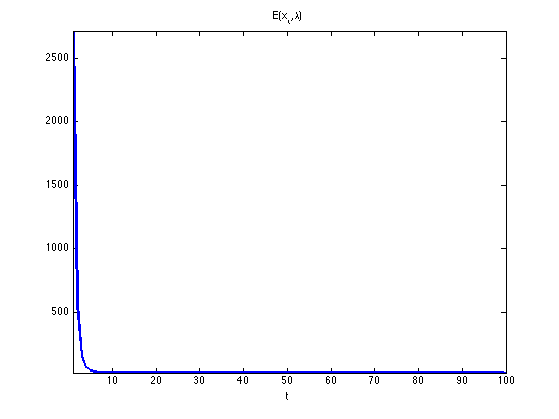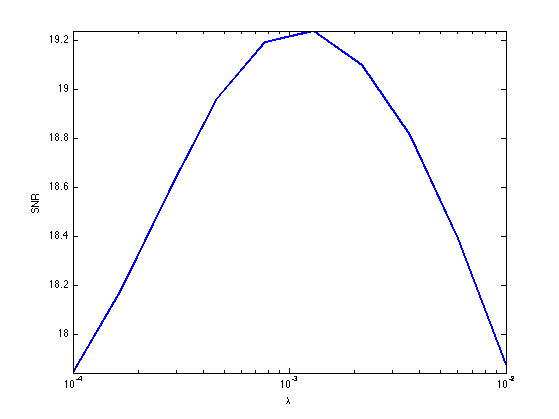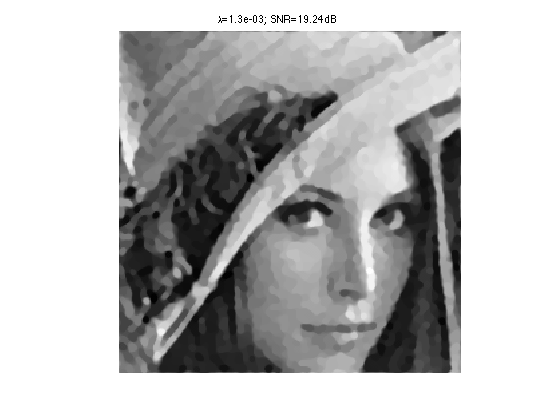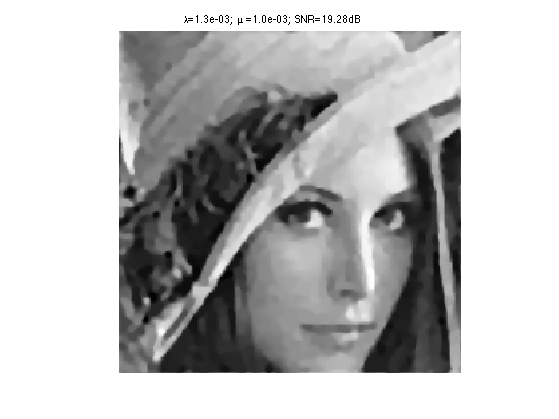$\newcommand{\NN}{\mathbb{N}} \newcommand{\CC}{\mathbb{C}} \newcommand{\GG}{\mathbb{G}} \newcommand{\LL}{\mathbb{L}} \newcommand{\PP}{\mathbb{P}} \newcommand{\QQ}{\mathbb{Q}} \newcommand{\RR}{\mathbb{R}} \newcommand{\VV}{\mathbb{V}} \newcommand{\ZZ}{\mathbb{Z}} \newcommand{\FF}{\mathbb{F}} \newcommand{\KK}{\mathbb{K}} \newcommand{\UU}{\mathbb{U}} \newcommand{\EE}{\mathbb{E}} \newcommand{\Aa}{\mathcal{A}} \newcommand{\Bb}{\mathcal{B}} \newcommand{\Cc}{\mathcal{C}} \newcommand{\Dd}{\mathcal{D}} \newcommand{\Ee}{\mathcal{E}} \newcommand{\Ff}{\mathcal{F}} \newcommand{\Gg}{\mathcal{G}} \newcommand{\Hh}{\mathcal{H}} \newcommand{\Ii}{\mathcal{I}} \newcommand{\Jj}{\mathcal{J}} \newcommand{\Kk}{\mathcal{K}} \newcommand{\Ll}{\mathcal{L}} \newcommand{\Mm}{\mathcal{M}} \newcommand{\Nn}{\mathcal{N}} \newcommand{\Oo}{\mathcal{O}} \newcommand{\Pp}{\mathcal{P}} \newcommand{\Qq}{\mathcal{Q}} \newcommand{\Rr}{\mathcal{R}} \newcommand{\Ss}{\mathcal{S}} \newcommand{\Tt}{\mathcal{T}} \newcommand{\Uu}{\mathcal{U}} \newcommand{\Vv}{\mathcal{V}} \newcommand{\Ww}{\mathcal{W}} \newcommand{\Xx}{\mathcal{X}} \newcommand{\Yy}{\mathcal{Y}} \newcommand{\Zz}{\mathcal{Z}} \newcommand{\al}{\alpha} \newcommand{\la}{\lambda} \newcommand{\ga}{\gamma} \newcommand{\Ga}{\Gamma} \newcommand{\La}{\Lambda} \newcommand{\Si}{\Sigma} \newcommand{\si}{\sigma} \newcommand{\be}{\beta} \newcommand{\de}{\delta} \newcommand{\De}{\Delta} \renewcommand{\phi}{\varphi} \renewcommand{\th}{\theta} \newcommand{\om}{\omega} \newcommand{\Om}{\Omega} \renewcommand{\epsilon}{\varepsilon} \newcommand{\Calpha}{\mathrm{C}^\al} \newcommand{\Cbeta}{\mathrm{C}^\be} \newcommand{\Cal}{\text{C}^\al} \newcommand{\Cdeux}{\text{C}^{2}} \newcommand{\Cun}{\text{C}^{1}} \newcommand{\Calt}{\text{C}^{#1}} \newcommand{\lun}{\ell^1} \newcommand{\ldeux}{\ell^2} \newcommand{\linf}{\ell^\infty} \newcommand{\ldeuxj}{{\ldeux_j}} \newcommand{\Lun}{\text{\upshape L}^1} \newcommand{\Ldeux}{\text{\upshape L}^2} \newcommand{\Lp}{\text{\upshape L}^p} \newcommand{\Lq}{\text{\upshape L}^q} \newcommand{\Linf}{\text{\upshape L}^\infty} \newcommand{\lzero}{\ell^0} \newcommand{\lp}{\ell^p} \renewcommand{\d}{\ins{d}} \newcommand{\Grad}{\text{Grad}} \newcommand{\grad}{\text{grad}} \renewcommand{\div}{\text{div}} \newcommand{\diag}{\text{diag}} \newcommand{\pd}{ \frac{ \partial #1}{\partial #2} } \newcommand{\pdd}{ \frac{ \partial^2 #1}{\partial #2^2} } \newcommand{\dotp}{\langle #1,\,#2\rangle} \newcommand{\norm}{|\!| #1 |\!|} \newcommand{\normi}{\norm{#1}_{\infty}} \newcommand{\normu}{\norm{#1}_{1}} \newcommand{\normz}{\norm{#1}_{0}} \newcommand{\abs}{\vert #1 \vert} \newcommand{\argmin}{\text{argmin}} \newcommand{\argmax}{\text{argmax}} \newcommand{\uargmin}{\underset{#1}{\argmin}\;} \newcommand{\uargmax}{\underset{#1}{\argmax}\;} \newcommand{\umin}{\underset{#1}{\min}\;} \newcommand{\umax}{\underset{#1}{\max}\;} \newcommand{\pa}{\left( #1 \right)} \newcommand{\choice}{ \left\{ \begin{array}{l} #1 \end{array} \right. } \newcommand{\enscond}{ \left\{ #1 \;:\; #2 \right\} } \newcommand{\qandq}{ \quad \text{and} \quad } \newcommand{\qqandqq}{ \qquad \text{and} \qquad } \newcommand{\qifq}{ \quad \text{if} \quad } \newcommand{\qqifqq}{ \qquad \text{if} \qquad } \newcommand{\qwhereq}{ \quad \text{where} \quad } \newcommand{\qqwhereqq}{ \qquad \text{where} \qquad } \newcommand{\qwithq}{ \quad \text{with} \quad } \newcommand{\qqwithqq}{ \qquad \text{with} \qquad } \newcommand{\qforq}{ \quad \text{for} \quad } \newcommand{\qqforqq}{ \qquad \text{for} \qquad } \newcommand{\qqsinceqq}{ \qquad \text{since} \qquad } \newcommand{\qsinceq}{ \quad \text{since} \quad } \newcommand{\qarrq}{\quad\Longrightarrow\quad} \newcommand{\qqarrqq}{\quad\Longrightarrow\quad} \newcommand{\qiffq}{\quad\Longleftrightarrow\quad} \newcommand{\qqiffqq}{\qquad\Longleftrightarrow\qquad} \newcommand{\qsubjq}{ \quad \text{subject to} \quad } \newcommand{\qqsubjqq}{ \qquad \text{subject to} \qquad }$

Generalized Forward-Backward Proximal Splitting

# Generalized Forward-Backward Proximal Splitting

This tour explores the use of an advanced non-smooth optimization scheme to handle composite inverse problems resolution.

## Contents

This tour is written by Hugo Raguet.

We use a proximal splitting algorithm detailed in

Hugo Raguet, Jalal M. Fadili and Gabriel Peyre, Generalized Forward-Backward Splitting Algorithm, preprint arXiv:1108.4404v2, 2011.

## Installing toolboxes and setting up the path.

You need to download the following files: signal toolbox and general toolbox.

You need to unzip these toolboxes in your working directory, so that you have toolbox_signal and toolbox_general in your directory.

For Scilab user: you must replace the Matlab comment '%' by its Scilab counterpart '//'.

Recommandation: You should create a text file named for instance numericaltour.sce (in Scilab) or numericaltour.m (in Matlab) to write all the Scilab/Matlab command you want to execute. Then, simply run exec('numericaltour.sce'); (in Scilab) or numericaltour; (in Matlab) to run the commands.

Execute this line only if you are using Matlab.

getd = @(p)path(p,path); % scilab users must *not* execute this


Then you can add the toolboxes to the path.

getd('toolbox_signal/');
getd('toolbox_general/');


## Convex Optimization with Generalized Forward-Backward Splitting

We consider general optimization problems of the form $\umin{x} F(x) + \sum_{i=1}^n G_i(x)$ where $$F$$ is a convex, differentiable function, with Lipschitz-continuous gradient and the $$G_i$$'s are convex functions

To use proximal algorithm, one should be able to compute the proximity operator of the $$G_i$$'s, defined as: $\text{prox}_{\gamma G_i}(x) = \uargmin{y} \frac{1}{2} \norm{x-y}^2 + \gamma G_i(y).$

The algorithm reads: $\text{for all } i, \quad z_{i,t+1} = z_{i,t} + \text{prox}_{n \gamma G_i}( 2 x_t - z_{i,t} - \gamma \nabla F(x_t) ) - x_t$ $x_{t+1} = \frac{1}{n} \sum_{i=1}^n z_{i,t+1}.$

It can be shown that if $$0 < \gamma < 2 \beta$$ where $$\frac{1}{\beta}$$ is a Lipschitz constant of $$\nabla F$$, then $${(x_t)}_t$$ converges to a minimizer of $$F + \sum_{i=1}^n G_i$$.

## Joint Inpainting and Deblurring

We consider a linear imaging operator $$\Phi : f \mapsto \Phi(f)$$ that maps high resolution images to low dimensional observations. Here we consider a composition of a pixel masking operator $$M$$ and of a blurring operator $$K$$.

Load an image $$f_0$$.

name = 'lena';
N = 256;
f0 = load_image(name);
f0 = rescale(crop(f0,N));


Display it.

clf
imageplot(f0);First, we define the masking operator. It is a projector on the set of valid pixels. It is equivalently implemented as a diagonal operator that multiplies the image by a binary mask

rho_M = .7;
mask = rand(N,N) > rho_M;
M = @(f) mask.*f;


Then, we define the blurring operator $$K$$, which is is a convolution with a kernel $$k$$: $$K(f) = k \star f$$.

We load a gaussian kernel $$k$$ of variance $$\si_K=2$$. Note that the Young's inequality gives for any $$f$$ $\norm{f \star k}_2 \leq \norm{f}_2 \norm{k}_1$ We normalize $$k$$ so that $$\norm{k}_1=1$$ which ensures $$\norm{K}=1$$.

sig_K = 2;
[X,Y] = meshgrid( [0:N/2-1 -N/2:-1] );
k = exp( - (X.^2+Y.^2) / (2*sig_K^2) );
k = k./sum(abs(k(:)));


A convolution is equivalent to a multiplication in the Fourier domain. $\Ff(f \star k) = \Ff(f) \cdot \Ff(k), \quad\text{so that}\quad K(f) = \Ff^{-1}(\Ff(f) \cdot \Ff(k))$ where $$\Ff$$ is the 2-D Fourier transform.

We thus implement $$K$$ using the Fourier transform of the kernel.

Fk = fft2(k);
K = @(f) real( ifft2( fft2(f).*Fk ) );


The masking and blurring operator $$\Phi = M \circ K$$.

Phi = @(f)M(K(f));


Compute the observations $$y = \Phi f_0 + w$$, where $$w$$ is a Gaussian white noise of variance $$\si_w$$.

sig_w = .025;
y = Phi(f0) + sig_w*randn(N,N);


Display it.

clf
imageplot(y);## Splitting Total Variation Regularization

We want to solve the noisy inverse problem $$y = \Phi x + w$$ using a total variation regularization: $\umin{x} \frac{1}{2} \norm{y - \Phi x}^2 + \la \norm{x}_{\text{TV}}.$

The total variation pseudo-norm is defined as the sum over all pixels $$p=(p_1,p_2)$$ of the norm of the image gradient $$\text{grad}(x)_p$$ at $$p$$.

The gradient is computed using finite differences $\text{grad} \, : \, \choice{ \mathbb{R}^{N \times N} \rightarrow \mathbb{R}^{N \times N \times 2} \\ x \mapsto ( x \star h_1, x \star h_2 ), }$ where $$h_1$$ and $$h_2$$ are two 2-D filters.

One usually computes the gradient using finite differences along vertical and horizontal directions. This corresponds to convolutions $$\text{grad}(x) = (x \star h_1,x \star h_2) \in \RR^{N \times N \times 2}$$ where $h_1 = \begin{pmatrix} 0 & 0 & 0 \\ 0 & -1 & 0 \\ 0 & 1 & 0 \end{pmatrix} \qandq h_2 = \begin{pmatrix} 0 & 0 & 0 \\ 0 & -1 & 1 \\ 0 & 0 & 0 \end{pmatrix}.$ Note that we write 2-D filters as $$3 \times 3$$ matrices, which implicitely assumes that the central index (position 0) is at the center of this matrix.

Following the method introduced in:

P. L. Combettes and J.-C. Pesquet, A proximal decomposition method for solving convex variational inverse problems, Inverse Problems, vol. 24, no. 6, article ID 065014, 27 pp., December 2008.

we use diagonal filters: $h_1 = \begin{pmatrix} 0 & 0 & 0 \\ 0 & -1 & 0 \\ 0 & 0 & 1 \end{pmatrix} \qandq h_2 = \begin{pmatrix} 0 & 0 & 0 \\ 0 & 0 & 1 \\ 0 &-1 & 0 \end{pmatrix}$ is more interesting for us, since those kernels are orthogonals: they do not overlap. We will use these diagonal filters to split the total variation into simpler functions.

The TV norm can be written as the $$\ell_1-\ell_2$$ norm $$G(u)$$ of the gradient $$u=\text{grad}(x)$$ $\norm{x}_{\text{TV}} = G( \text{grad}(x) ) \qwhereq G(u) = \sum_{p} \norm{u_p} = \sum_p \sqrt{ {u_{1,p}}^2 + {u_{2,p}}^2 }$ where $$u = ( (u_{1,p},u_{2,p}) )_{p} \in \RR^{N \times N \times 2}$$ is a vector field.

The proximal operator of $$G$$ is the soft thresholding of the norm of each vector $$u_p \in \RR^2$$ in the vector field, which corresponds to $\text{prox}_{\gamma G}(u)_{p} = \max\pa{0, 1-\frac{\gamma}{\norm{u_{p}}}} u_{p} .$ If the norm of $$u_{p}$$ is lower than $$\gamma$$, then $$u_p$$ is set to zero; otherwise, both coordinates of $$u_p$$ are shrinked by the same factor.

We define the proximity operator of $$G$$.

G = @(u) sqrt( sum( u.^2, 3 ) );
proxG = @(u,gamma) repmat( max(0,1 - gamma./G(u) ), [1 1 2] ).*u;


We would like to compute the proximity operator of $$\norm{\cdot}_{\text{TV}} = G \circ \text{grad}$$. This is much more complicated than computing the proximity operator of $$G$$ because the linear operator $$\text{grad}$$ introduces dependancies between pixels.

Denoting $$u=\text{grad}(x)=(u_{1,p},u_{2,p})_{p=(p_1,p_2)}$$ the gradient vector, we note that we can split the TV pseudo norm according to the parity of $$p_1=2r_1+s_1$$ and $$p_2=2r_2+s_2$$ (which corresponds to $$s_i$$ being either 0 or 1) $\norm{x}_{\text{TV}} = \sum_{s_1,s_2=0,1} \sum_{(r_1,r_2)} \sqrt{ {u_{1,2 r_1+s_1,2r_2+s_2}}^2 + {u_{2,2 r_1+s_1,2r_2+s_2}}^2 }.$

This can be re-written more compactly as a split of the TV pseudo-norm using using $$n=4$$ simple functions $$(G_i)_{i=1}^4$$: $\norm{x}_{\text{TV}} = \sum_{i=1}^{4} G_i(x) \qwhereq \choice{ G_i = G \circ L_i, \\ L_i = S \circ \text{grad} \circ T_{s^{(i)}} }$ where $$T_s$$ is the shifting operator that translates the pixels by $$s=(s_1,s_2)$$ (with periodic boundary condition, i.e. modulo $$N$$) $T_s(x)_p = x_{p_1-s_1 \text{ mod } N, p_2-s_2 \text{ mod } N}$ and $$S : \RR^{N \times N} \rightarrow \RR^{N/2 \times N/2}$$ is the sub-sampling operator of a vector field by a factor of two along vertical and horizontal directions $S(u)_{p_1,p_2} = u_{2p_1,2p_2}.$ The four shifts are $s \in \{ (0,0), (1,0), (0,1), (1,1) \} .$

We create the subsampled gradient operator $$L_1 = S \circ \text{grad}$$ (shift $$s =(0,0)$$).

L = @(x) cat( 3, x(2:2:end,2:2:end)-x(1:2:end,1:2:end), x(1:2:end,2:2:end)-x(2:2:end,1:2:end) );


We create the four shifted version $$L_1,L_2,L_3,L_4$$ and store them using a cell array, so that Li{i} implements $$L_i$$.

LShift = @(x,s) L( circshift(x,s) );
Li = {@(x)LShift(x,[0,0]), @(x)LShift(x,[1,0]), @(x)LShift(x,[0,1]), @(x)LShift(x,[1,1]) };


Define the four functionals $$(G_i)_{i=1}^4$$.

for i=1:4
Gi{i} = @(x) sum( sum( G( Li{i}(x) ) ) );
end


Since $$L_1 = S \circ \text{grad}$$ its ajoint reads $$L_1^* = \text{grad}^* \circ U$$ where $$U$$ is the upsampling operator $U(v)_{2p_1+s_1,2p_2+s_2} = \choice{ v_{p_1,p_2} \qifq s_1=s_2=0, \\ 0 \quad \text{otherwise}. }$

U = @(x)upsampling( upsampling( x, 1, 2 ), 2, 2 );


The adjoint of the gradient $$\text{grad}^* =-\text{div}$$ $\text{grad}^*(u) = u_1 \star \bar h_1 + u_2 \star \bar h_2 \in \RR^{N \times N}$ is obtained using the reversed filters $\bar h_1 = \begin{pmatrix} 1 & 0 & 0 \\ 0 & -1 & 0 \\ 0 & 0 & 0 \end{pmatrix} \qandq \bar h_2 = \begin{pmatrix} 0 &-1 & 0 \\ 1 & 0 & 0 \\ 0 & 0 & 0 \end{pmatrix}$

revIdx = [N 1:N-1];
gradS = @(u)(u(revIdx,revIdx,1) - u(:,:,1)) + (u(:,revIdx,2) - u(revIdx,:,2));


Define the adjoint operators of $$L_1$$.

L1S = @(v)gradS(U(v));


Define the adjoint $$L_{i}^*$$ of $$L_{i}$$. Since $$L_i = L_1 \circ T_{s_i}$$, one has $$L_i^* = T_{s_i}^* \circ L_i^* = T_{-s_i} \circ L_i^*$$.

LShiftS = @(gx,s) circshift( L1S( gx ), -s );
LiS = { @(x)LShiftS(x,[0,0]), @(x)LShiftS(x,[1,0]), @(x)LShiftS(x,[0,1]), @(x)LShiftS(x,[1,1]) };


Computing the finite differences with above mentioned orthogonal kernels implies the crucial property that the four operators $L_i : \mathbb{R}^{N \times N} \mapsto \mathbb{R}^{\frac{N}{2} \times \frac{N}{2} \times 2}$ are tight frames, i.e. satisfy $L_i \circ L_i^* = b \, \text{Id}$ for some $$b \in \RR$$.

Exercice 1: (check the solution) Check that each subsampled gradient $$L_i$$ is indeed a tight frame, and determine the value of $$b$$. You can for instance apply the operators to random vector fields.

exo1;


We are now ready to compute the proximity operators of each $$G_i$$. Recall that rules of proximal calculus gives us that if $$L \circ L^* = b \, \text{Id}$$, then $\text{prox}_{G \circ L} = \text{Id} + \frac{1}{b} L^* \circ \left( \text{prox}_{b G} - \text{Id} \right) \circ L$

Create the proximity operator of $$G_i$$.

proxG_Id = @(u,gamma)proxG(u,gamma) - u;
proxGi = @(x,gamma,i)x + (1/b)*LiS{i}( proxG_Id( Li{i}(x), b*gamma ) );


## Using Smoothness of Data-Fidelity

We rewrite the initial optimization problem as $\umin{x} E(x,\la) = F(x) + \la \sum_{i=1}^4 G_i(x)$ where the data-fidelity term is $F(x) = \frac{1}{2} \norm{y - \Phi x}^2 .$

F = @(x) (1/2)*sum( sum( (Phi(x) - y).^2 ) );
E = @(x,lambda) F(x) + lambda * ( Gi{1}(x) + Gi{2}(x) + Gi{3}(x) + Gi{4}(x) );


Computing the proximity operator of $$F$$ requires the resolution of a linear system. To avoid such a time-consuming task, the GFB makes use of the smoothness of $$F$$. Its gradient is $\nabla F(x) = \Phi^* (\Phi(x) - y).$

Important: be careful not to confuse $$\text{grad}(x)$$ (the gradient of the image) with $$\nabla F$$ (the gradient of the functional).

We define the adjoint operator $$\Phi^*$$ of $$\Phi$$. One has $$\Phi^* = K^* \circ M^* = K \circ M$$ since $$M^* = M$$ because it is an orthogonal projector and $$K^* = K$$ because it is a convolution with a symetric kernel.

Phis = @(f)K(M(f));


Create the gradient operator $$\nabla F$$.

nablaF = @(x)Phis( Phi(x) - y );


Moreover, $$\nabla F$$ is affine, so that it is Lipschitz-continuous with Lipschitz constant equal to the norm of its linear part $\frac{1}{\beta} = \norm{\Phi^* \circ \Phi} \leq \norm{M} \times \norm{K} = 1,$ since $$\norm{M} = 1$$ because it is a projector and $$\norm{K} = 1$$ because it is a convolution with a normalized kernel. Hence in the following we define $$\be = 1$$.

beta = 1;


## Solving with Generalized Forward-Backward

We are now ready to minimize the functional $$E(x,\la)$$.

Set the number of parts $$n$$ in the non-smooth splitting

n = 4;


Define the GFB step size $$\gamma$$ that should satisfy $$0 < \gamma < 2 \be$$.

gamma = 1.8*beta;


Choose a regularization parameter $$\la>0$$.

lambda = 1e-4;


Exercice 2: (check the solution) The parameter $$\la$$ does not appear explicitely in the iterations of the generalized forward-backward algorithm. Where does it step in ?

exo2;


The iterates $$x_t$$ of the GFB will be stored in a variable x that we initialize to $$x_0=0$$.

x = zeros(N,N);


The auxiliary variables $$z_{i,t}$$ will be stored in a $$N \times N \times n$$ array z initialized to 0, so that z(:,:,i) stores $$z_{i,t}$$.

z = zeros(N,N,n);


Exercice 3: (check the solution) Compute 100 iterations of the generalized forward-backward, while monitoring the value $$E(x_t,\la)$$ of the objective at iteration $$t$$. Display the evolution of the objective along iterations: it must decrease.

exo3;Now that we know how to minimize our functional, we must seek for the most relevent regularization parameter $$\la$$. Because we know the original image $$f_0$$, we can compare it to the recovered image $$x$$ for different values of $$\la$$. Use the signal-to-noise ratio (SNR) as criterium.

Define a range of acceptable values for $$\la$$.

lambdaList = logspace( -4, -2, 10 );


Exercice 4: (check the solution) Display the resulting SNR as a function of $$\la$$. Take the best regularization parameter and display the corresponding recovery.

exo4;Display the final image, that has been saved in the variable recov.

clf
imageplot(recov)
title( sprintf( '\\lambda=%.1e; SNR=%.2fdB', bestLambda, SNRmax ) );## Bonus: Composite Regularization

The degradation operator $$\Phi$$ is very aggressive. To achieve better recovery, it is possible to mix several priors. Let us add a wavelet analysis sparsity prior in the objective $\umin{x} F(x) + \la \sum_{i=1}^4 G_i(x) + \mu G_5(x) \qwhereq G_5(x) = \norm{\Psi x}_1$ where $$\Psi$$ is an orthogonal wavelet transform and $$\norm{\cdot}_1$$ is the $$\ell_1$$-norm.

The number $$n$$ of simple functionals is now 5.

n = 5;


Create the wavelet transform $$\Psi$$.

Jmin = 4;
Psi = @(x)perform_wavortho_transf(x,Jmin,+1);


Define its adjoint $$\Psi^*$$, where we use the fact that the adjoint of an othogonal operator is its inverse.

Psis = @(x)perform_wavortho_transf(x,Jmin,-1);


Similarly to the $$\ell_1-\ell_2$$-norm, the proximity operator of the $$\ell_1$$-norm is a soft-thresholding on each coefficients. Because $$\Psi$$ is orthogonal, it is also a tight frame operator with bound $$b=1$$.

Create the proximity operator of $$G_5$$.

l1norm = @(wx)abs(wx);
proxl1 = @(wx,gamma) max(0,1 - gamma./l1norm(wx) ).*wx;
proxG5 = @(x,gamma) Psis( proxl1(Psi(x),gamma) );


Exercice 5: (check the solution) Solve the composite regularization model. Keep the previous value of $$\la$$, set $$\mu = 10^{-3}$$, and perform 500 iterations. Display the results and compare visually to the previous one. Is the SNR significantly improved ? Conclude on the SNR as a quality criterium, and on the usefulness of mixing different regularizations priors.

exo5;